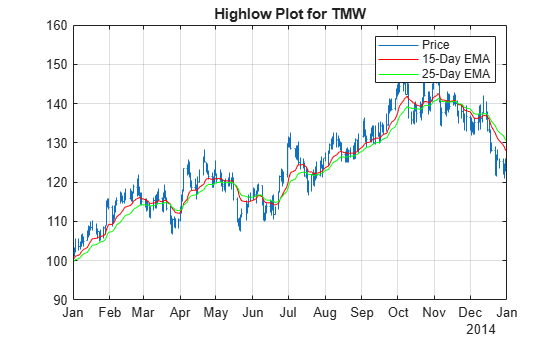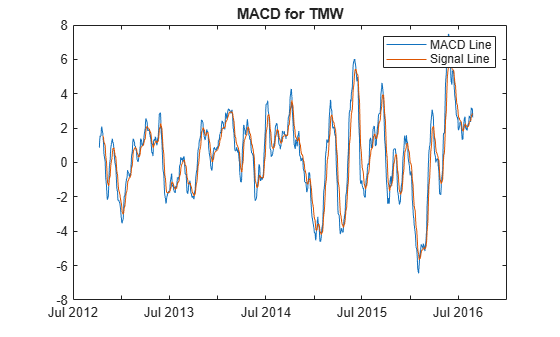Documentation

## Using Timetables in Finance

This example shows how to use timetables to visualize and calculate weekly statistics from simulated daily stock data.

The data for this example is in the MAT-file `SimulatedStock.mat`, which loads the following:

• Dates corresponding to the closing stock prices, `TMW_DATES`

• Opening stock prices, `TMW_OPEN`

• Daily high of stock prices, `TMW_HIGH`

• Daily low of stock prices, `TMW_LOW`

• Closing stock prices, `TMW_CLOSE, TMW_CLOSE_MISSING`

• Daily volume of traded, `TMW_VOLUME`

• Data in a table, `TMW_TB`

`load SimulatedStock.mat TMW_*`

Step 2. Create timetables.

In timetables, you can work with financial time series rather than with vectors. When using a `timetable`, you can easily track the dates. You can manipulate the data series based on the dates, because a `timetable` object tracks the administration of a time series.

Use the MATLAB® `timetable` function to create a `timetable` object. Alternatively, you can use the MATLAB conversion function `table2timetable` to convert a table to a timetable. In this example, the timetable `TMW_TT` is constructed from a table and is only for illustration purposes. After you create a `timetable` object, you can use the `Description` field of the `timetable` object to store meta-information about the timetable.

```% Create a timetable from vector input TMW = timetable(TMW_OPEN,TMW_HIGH,TMW_LOW,TMW_CLOSE_MISSING,TMW_VOLUME, ... 'VariableNames',{'Open','High','Low','Close','Volume'},'RowTimes',TMW_DATES); % Convert from a table to a timetable TMW_TT = table2timetable(TMW_TB,'RowTimes',TMW_DATES); TMW.Properties.Description = 'Simulated stock data.'; TMW.Properties```
```ans = TimetableProperties with properties: Description: 'Simulated stock data.' UserData: [] DimensionNames: {'Time' 'Variables'} VariableNames: {'Open' 'High' 'Low' 'Close' 'Volume'} VariableDescriptions: {} VariableUnits: {} VariableContinuity: [] RowTimes: [1000x1 datetime] StartTime: 04-Sep-2012 SampleRate: NaN TimeStep: NaN CustomProperties: No custom properties are set. Use addprop and rmprop to modify CustomProperties. ```

Step 3. Calculate basic data statistics, and fill the missing data.

Use the MATLAB `summary` function to view basic statistics of the `timetable` data. By reviewing the summary for each variable, you can identify missing values. You can then use the MATLAB `fillmissing` function to fill in missing data in a timetable by specifying a fill method.

```summaryTMW = summary(TMW); summaryTMW.Close```
```ans = struct with fields: Size: [1000 1] Type: 'double' Description: '' Units: '' Continuity: [] Min: 83.4200 Median: 116.7500 Max: 162.1100 NumMissing: 3 ```
```TMW = fillmissing(TMW,'linear'); summaryTMW = summary(TMW); summaryTMW.Close```
```ans = struct with fields: Size: [1000 1] Type: 'double' Description: '' Units: '' Continuity: [] Min: 83.4200 Median: 116.7050 Max: 162.1100 NumMissing: 0 ```
`summaryTMW.Time`
```ans = struct with fields: Size: [1000 1] Type: 'datetime' Min: 04-Sep-2012 Median: 31-Aug-2014 Max: 24-Aug-2016 NumMissing: 0 TimeStep: NaN ```

Step 4. Visualize the data.

To visualize the timetable data, use financial charting functions such as `highlow` or `movavg`. For this example, the moving average information is plotted on the same chart for `highlow` to provide a complete visualization. To obtain the stock performance in 2014, use the MATLAB `timerange` function to select rows of the `timetable`. To visualize a technical indicator such as the Moving Average Convergence Divergence (MACD), pass the `timetable` object into the `macd` function for analysis.

```index = timerange(datetime('01-Jan-2014','Locale','en_US'),datetime('31-Dec-2014','Locale','en_US'),'closed'); highlow(TMW(index,:)); hold on ema15 = movavg(TMW(:,'Close'),'exponential',15); ema25 = movavg(TMW(:,'Close'),'exponential',25); ema15 = ema15(index,:); ema25 = ema25(index,:); plot(ema15.Time,ema15.Close,'r'); plot(ema25.Time,ema25.Close,'g'); hold off legend('Price','15-Day EMA','25-Day EMA') title('Highlow Plot for TMW')``````[macdLine, signalLine] = macd(TMW(:,'Close')); plot(macdLine.Time,macdLine.Close); hold on plot(signalLine.Time,signalLine.Close); hold off title('MACD for TMW') legend('MACD Line', 'Signal Line')```Step 5. Create a weekly return and volatility series.

To calculate weekly return from the daily stock prices, you must resample the data frequency from daily to weekly. When working with timetables, use the MATLAB functions `retime` or `synchronize` with various aggregation methods to calculate weekly statistics. To adjust the timetable data to a time-vector basis, use `retime` and use `synchronize` with multiple timetables.

```weeklyOpen = retime(TMW(:,'Open'),'weekly','firstvalue'); weeklyHigh = retime(TMW(:,'High'),'weekly','max'); weeklyLow = retime(TMW(:,'Low'),'weekly','min'); weeklyClose = retime(TMW(:,'Close'),'weekly','lastvalue'); weeklyTMW = [weeklyOpen,weeklyHigh,weeklyLow,weeklyClose]; weeklyTMW = synchronize(weeklyTMW,TMW(:,'Volume'),'weekly','sum'); head(weeklyTMW)```
```ans=8×6 timetable Time Open High Low Close Volume ___________ ______ ______ ______ ______ __________ 02-Sep-2012 100 102.38 98.45 99.51 2.7279e+07 09-Sep-2012 99.72 101.55 96.52 97.52 2.8518e+07 16-Sep-2012 97.35 97.52 92.6 93.73 2.9151e+07 23-Sep-2012 93.55 98.03 92.25 97.35 3.179e+07 30-Sep-2012 97.3 103.15 96.68 99.66 3.3761e+07 07-Oct-2012 99.76 106.61 98.7 104.23 3.1299e+07 14-Oct-2012 104.54 109.75 100.55 103.77 3.1534e+07 21-Oct-2012 103.84 104.32 96.95 97.41 3.1706e+07 ```

To perform calculations on entries in a `timetable`, use the MATLAB `rowfun` function to apply a function to each row of a weekly frequency timetable.

```returnFunc = @(open,high,low,close,volume) log(close) - log(open); weeklyReturn = rowfun(returnFunc,weeklyTMW,'OutputVariableNames',{'Return'}); weeklyStd = retime(TMW(:,'Close'),'weekly',@std); weeklyStd.Properties.VariableNames{'Close'} = 'Volatility'; weeklyTMW = [weeklyReturn,weeklyStd,weeklyTMW] ```
```weeklyTMW=208×8 timetable Time Return Volatility Open High Low Close Volume ___________ ___________ __________ ______ ______ ______ ______ __________ 02-Sep-2012 -0.004912 0.59386 100 102.38 98.45 99.51 2.7279e+07 09-Sep-2012 -0.022309 0.63563 99.72 101.55 96.52 97.52 2.8518e+07 16-Sep-2012 -0.037894 0.93927 97.35 97.52 92.6 93.73 2.9151e+07 23-Sep-2012 0.039817 2.0156 93.55 98.03 92.25 97.35 3.179e+07 30-Sep-2012 0.023965 1.1014 97.3 103.15 96.68 99.66 3.3761e+07 07-Oct-2012 0.043833 1.3114 99.76 106.61 98.7 104.23 3.1299e+07 14-Oct-2012 -0.0073929 1.8097 104.54 109.75 100.55 103.77 3.1534e+07 21-Oct-2012 -0.063922 2.1603 103.84 104.32 96.95 97.41 3.1706e+07 28-Oct-2012 -0.028309 0.9815 97.45 99.1 92.58 94.73 1.9866e+07 04-Nov-2012 -0.00010566 1.224 94.65 96.1 90.82 94.64 3.5043e+07 11-Nov-2012 0.077244 2.4854 94.39 103.98 93.84 101.97 3.0624e+07 18-Nov-2012 0.022823 0.55896 102.23 105.27 101.24 104.59 2.5803e+07 25-Nov-2012 -0.012789 1.337 104.66 106.02 100.85 103.33 3.1402e+07 02-Dec-2012 -0.043801 0.2783 103.37 103.37 97.69 98.94 3.2136e+07 09-Dec-2012 -0.063475 1.9826 99.02 99.09 91.34 92.93 3.4447e+07 16-Dec-2012 0.0025787 1.2789 92.95 94.2 88.58 93.19 3.3247e+07 ⋮ ```